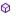Press on the image to return to the main documentation page.

### Written by Derez

#### List of types:

The library provide several methods which can be used

None

#### Members:FlatArea (UTMlist As java.util.List) As DoubleFlatArea2 (Poligon(,) As Double, n As Int, UindxX As Int, UindxY As Int) As DoubleGeoDistanceBearing (Lat1 As Double, Long1 As Double, Lat2 As Double, Long2 As Double) As Double()GeoRouteLength (GEOlist As java.util.List) As DoubleGeoRouteLength2 (Poligon(,) As Double, start As Int, last As Int, GindxLat As Int, GindxLong As Int) As DoubleLatLonToUTM (a As Double, f As Double, Lat As Double, Lon As Double) As Double()SphericalArea (GEOlist As java.util.List) As DoubleSphericalArea2 (Poligon(,) As Double, n As Int, GindxLat As Int, GindxLong As Int) As DoubleUTMDistanceBearing (x1 As Double, y1 As Double, x2 As Double, y2 As Double) As Double()UTMRouteLength (UTMlist As java.util.List) As DoubleUTMRouteLength2 (Poligon(,) As Double, start As Int, last As Int, UindxX As Int, UindxY As Int) As DoubleUTMToLatLon (a As Double, f As Double, UtmXZone As Int, Easting As Double, NorthHemisphere As Boolean, Northing As Double) As Double()

#### Members description:

#####FlatArea (UTMlistAsjava.util.List) AsDouble
The methods calculates the area enclosed inside a polygon which is defined in the list parameter.
Every list item should be a string of the form "UTMX,UTMY".
The returned area is in square Km.
#####FlatArea2 (Poligon(,) AsDouble, nAsInt, UindxXAsInt, UindxYAsInt) AsDouble
The methods calculates the area enclosed inside a polygon.
n is the polygon's size.
UindxX and UindxY are the indices of the parameters UTMx and UTMy in the array.
The returned area is in square Km.
#####GeoDistanceBearing (Lat1AsDouble, Long1AsDouble, Lat2AsDouble, Long2AsDouble) AsDouble()
Calculates the Distance in Km and Bearing in degrees, from point 1 to 2.
The calculated "straight line" is a Great Arc which is the shortest on a sphere.
#####GeoRouteLength (GEOlistAsjava.util.List) AsDouble
For a list of GEO points, the method calculates
the length of the total route in Km.
Every list item should be a string of the form "Lat,Long".
#####GeoRouteLength2 (Poligon(,) AsDouble, startAsInt, lastAsInt, GindxLatAsInt, GindxLongAsInt) AsDouble
For an array of GEO points, the method calculates
the length of a route which starts at point "start" and ends at "last".
GindxLat and GindxLong are the indices of the parameters Lat and Long in the array.
The result is in Km.
#####LatLonToUTM (aAsDouble, fAsDouble, LatAsDouble, LonAsDouble) AsDouble()
This function converts the specified lat/lon coordinate to a UTM coordinate.
Parameters:
double a: Ellipsoid semi-major axis, in meters.
(For WGS84 datum, use 6378137.0)
double f: Ellipsoid flattening.
(For WGS84 datum, use 1 / 298.257223563).
int utmXZone:
Upon exit, this parameter will contain the hotizontal
zone number of the UTM coordinate.
The returned value for this parameter is a number
within the range 1 to 60, inclusive.
char utmYZone: Upon exit, this parameter will contain
the zone letter of the UTM coordinate.
The returned value for this parameter will be one of:
CDEFGHJKLMNPQRSTUVWX.
double easting: Upon exit, this parameter will contain
the UTM easting, in meters.
double northing: Upon exit, this parameter will contain
the UTM northing, in meters.
double lat, double lon: The lat/lon coordinate to convert.
The returned double array is in this order:
easting, northing ,utmXZone, utmYzone.
#####SphericalArea (GEOlistAsjava.util.List) AsDouble
The methods calculates the area on the Earth's spherical enclosed inside a polygon
which is defined in the list parameter.
Every list item should be a string of the form "Lat,Long".
The returned area is in square Km.
#####SphericalArea2 (Poligon(,) AsDouble, nAsInt, GindxLatAsInt, GindxLongAsInt) AsDouble
The methods calculates the area on the Earth's spherical enclosed inside a polygon.
n is the polygon's size.
GindxLat and GindxLong are the indices of the parameters Lat and Long in the array.
The returned area is in square Km.
#####UTMDistanceBearing (x1AsDouble, y1AsDouble, x2AsDouble, y2AsDouble) AsDouble()
Calculates the Distance in Km and Bearing in degrees,
from point 1 to 2.
The calculation is done as if the area is flat.
#####UTMRouteLength (UTMlistAsjava.util.List) AsDouble
For a list of UTM points, the method calculates
the length of the total route in Km.
Every list item should be a string of the form "UTMX,UTMY".
#####UTMRouteLength2 (Poligon(,) AsDouble, startAsInt, lastAsInt, UindxXAsInt, UindxYAsInt) AsDouble
For an array of UTM points, the method calculates
the length of a route which starts at point "start" and ends at "last".
UindxX and UindxY are the indices of the parameters UTMx and UTMy in the array.
The result is in Km.
#####UTMToLatLon (aAsDouble, fAsDouble, UtmXZoneAsInt, EastingAsDouble, NorthHemisphereAsBoolean, NorthingAsDouble) AsDouble()
This function converts the specified UTM coordinate to a lat/lon
coordinate.
- utmXZone must be between 1 and 60, inclusive.
- utmYZone must be one of: CDEFGHJKLMNPQRSTUVWX
Parameters:
double a: Ellipsoid semi-major axis, in meters.
(For WGS84 datum, use 6378137.0)
double f: Ellipsoid flattening. (For WGS84 datum, use 1 / 298.257223563)
int utmXZone: The horizontal zone number of the UTM coordinate.
char utmYZone: The vertical zone letter of the UTM coordinate.
double easting, double northing: The UTM coordinate to convert.
double lat: Upon exit, lat contains the latitude.
double lon: Upon exit, lon contains the longitude.
Top